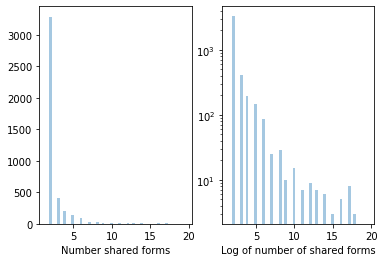# The long tail of federal form elements¶

Examining the distribution of shared data elements across forms.

In :
import xml.etree.ElementTree as et
import matplotlib.pyplot as plt
import seaborn as sns

# Data downloaded from https://repository.usaspending.gov/cder_library/authorized/report/common
with open('/content/CDER_Library_DataElements_202001261038.xml','r') as fp:
tree = et.parse(fp)

In :
# Examining the number of data elements total
d = tree.getroot()
print(f"There are {len(d)} data elements that appear on 2 or more forms.")

There are 4278 data elements that appear on 2 or more forms.

In :
# Creating a list of the number of times that each element appears on a form
common_list = [len(elem.findall(".//form")) for elem in d]

In :
# Plotting the histogram
# Note: I chose to plot the histogram *and* the log of the histogram to demonstrate that the long tail has some texture
fig, axs = plt.subplots(ncols=2)
sns.distplot(common_list, hist=True, hist_kws={"range":(1.5,19.5),"log":False}, kde=False, norm_hist=False, axlabel="Number shared forms", ax=axs)
sns.distplot(common_list, hist=True, hist_kws={"range":(1.5,19.5),"log":True}, kde=False, norm_hist=False, axlabel="Log of number of shared forms", ax=axs)

Out:
<matplotlib.axes._subplots.AxesSubplot at 0x7fe7085f4cc0>